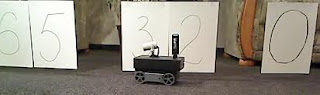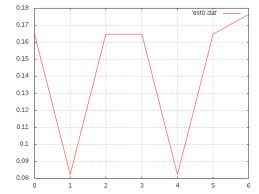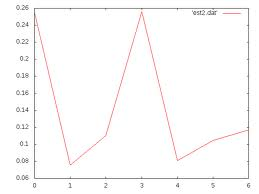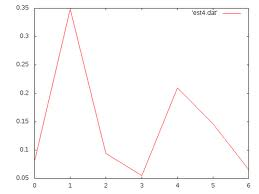### One-dimensional robot localization example

Here is my first example for the Unit 1 of the CS373 free online class. Please see this post for the introduction of this example series. This example illustrates how to perform one-dimensional localization using histogram filter we have learned in Unit 1 of the class. I will be using our Veter vehicle to illustrate how to control real robotics vehicle. To illustrate the one-dimensional localization algorithm, I have made the following test environment:Figure 1: Test set up for the one-dimensional localization test

The Figure 1 illustrates our vehicle and the line of A4 paper sheets which represents the "wall". Each sheet is labeled with the number (0, 2, 3, etc., from right to left) which is the position within the line. Sheets with numbers 1 and 4 are removed to simulate the "door". We assume here, that robot can make discrete movement one position left or right. So the localization problem is to determine current position of the robot, i.e. in front of which sheet the robot currently is. So it roughly corresponds to the following situation :-) :I hope I am not violating any copyrights here by re-posting this picture from Unit 1 Notes. Otherwise, please let me know and I will remove it.
For demonstration purposes, we decide to perform measurements using on-board ultrasonic range finder (aka. sonar). Since our robot has only one sonar on the front panel, we need to turn towards the "wall" (or "door", we do not know...) to make measurements, then turn back and drive forward to the next adjustment position. We assume, that we start at the arbitrary unknown position facing towards the wall. So the motion step is: turn left, drive forward, turn right back to the wall, query and process new measurement. For simplicity, I assume that if sonar shows distance less then 50cm, than we are in front of wall. Otherwise, if distance is bigger, then there is nothing in front of us, i.e. we are facing the door.

To perform precise motions, like for example turning exactly 90 degrees, additional sensor data required (for example compass) as well as control algorithms such as PID or whatever else. However, to keep this example as simple as possible and make sure that focus stays on the localization topic, I decide to implement the control in very naive way. I turn corresponding motors on, wait some period of time and then turn them off. For example, turning the left track clockwise and the right counter-clockwise will rotate the robot to the left. So I just measure the time needed to make ~90 degrees turn and turning motors off after this amount of time. It works only for concrete surface, varies when battery gets discharged and very imprecise. But again, it was not a purpose of this example and I want to keep it as simple as possible. There is control algorithms topic in the course syllabus, so hopefully I will be able to address issues mentioned above with following examples for the corresponding units.

The whole program, which is in localization.py, defines two classes SensorDataReceiverI which is a callback interface to receive sensor data pushed from the vehicle. The nextSensorFrame() method is invoked every time the new sensor data arrives. I store just the last received measurement for compass and sonar to read them later. The second class is Client which is derived from Ice.Application class. Client class defines sense() and move() methods which perform sensor data and movement processing steps of the localization algorithm. They are copy/pasted from the Unit1 lecture. The run() method is the application entry point. It connects to the remote vehicle, set up the callback interface and then executes five sense/move steps to update position estimation probabilities. In addition, it also invoke corresponding commands to control the vehicle. In particular, there is a function makeMotionStep() defined in the Client class to perform turn-left/move/drive-forward/turn-right motion sequence mentioned above. Finally, for each step, the location probability array is printed out.

All sources are available on GitHub. .ice files over there are interface definition for remote communication. They will be automatically processed by Ice.loadSlice("--all vehicle.ice") command. More details on how to use ICE middleware could be found here. Documentation for the Python language binding for ICE is available here.

The following video shows the test set-up and robotics vehicle made five motion steps.

Finally, the set of graphs below illustrate the position estimation (probability of being at certain position) for each of five steps. This graphs are made based on the output produced by the python control program described above. It is easy to see, that after the step 5, there is much higher probability of being at the second position from the left then somewhere else. So, we solve the localization problem.Step 1Step 2Step3Step 4Step 5